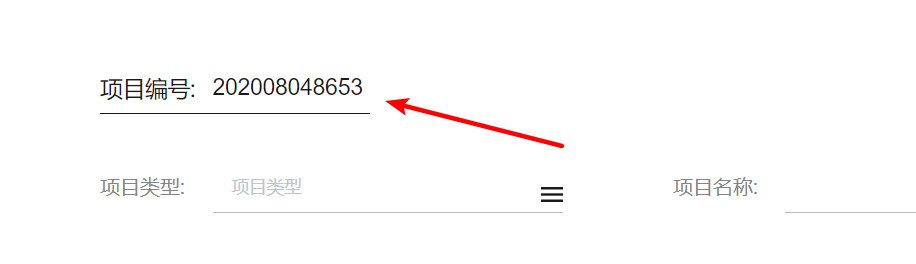``````created () {
// 调用获取当前日期的方法加四位随机数  赋值表单中的项目编号
this.form.number = this.getProjectNum() + Math.floor(Math.random() * 10000)  // 如果是6位或者8位随机数，相应的 *1000000或者 *100000000就行了
},
methods: {
// 获取当前日期的方法
getProjectNum () {
const projectTime = new Date() // 当前中国标准时间
const Year = projectTime.getFullYear() // 获取当前年份 支持IE和火狐浏览器.
const Month = projectTime.getMonth() + 1 // 获取中国区月份
const Day = projectTime.getDate() // 获取几号
var CurrentDate = Year
if (Month >= 10) { // 判断月份和几号是否大于10或者小于10
CurrentDate += Month
} else {
CurrentDate += '0' + Month
}
if (Day >= 10) {
CurrentDate += Day
} else {
CurrentDate += '0' + Day
}
return CurrentDate
}
}
``````